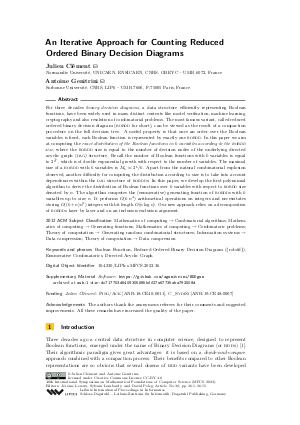Document# An Iterative Approach for Counting Reduced Ordered Binary Decision Diagrams

### Authors Julien Clément, Antoine Genitrini## File

LIPIcs.MFCS.2023.36.pdf
• Filesize: 0.92 MB
• 15 pages

## Acknowledgements

The authors thank the anonymous referees for their comments and suggested improvements. All these remarks have increased the quality of the paper.

## Cite As

Julien Clément and Antoine Genitrini. An Iterative Approach for Counting Reduced Ordered Binary Decision Diagrams. In 48th International Symposium on Mathematical Foundations of Computer Science (MFCS 2023). Leibniz International Proceedings in Informatics (LIPIcs), Volume 272, pp. 36:1-36:15, Schloss Dagstuhl - Leibniz-Zentrum für Informatik (2023)
https://doi.org/10.4230/LIPIcs.MFCS.2023.36

## Abstract

For three decades binary decision diagrams, a data structure efficiently representing Boolean functions, have been widely used in many distinct contexts like model verification, machine learning, cryptography and also resolution of combinatorial problems. The most famous variant, called reduced ordered binary decision diagram (robdd for short), can be viewed as the result of a compaction procedure on the full decision tree. A useful property is that once an order over the Boolean variables is fixed, each Boolean function is represented by exactly one robdd. In this paper we aim at computing the {exact distribution of the Boolean functions in k variables according to the robdd size}, where the robdd size is equal to the number of decision nodes of the underlying directed acyclic graph (dag) structure. Recall the number of Boolean functions with k variables is equal to 2^{2^k}, which is of double exponential growth with respect to the number of variables. The maximal size of a robdd with k variables is M_k ≈ 2^k / k. Apart from the natural combinatorial explosion observed, another difficulty for computing the distribution according to size is to take into account dependencies within the dag structure of robdds. In this paper, we develop the first polynomial algorithm to derive the distribution of Boolean functions over k variables with respect to robdd size denoted by n. The algorithm computes the (enumerative) generating function of robdds with k variables up to size n. It performs O(k n⁴) arithmetical operations on integers and necessitates storing O((k+n) n²) integers with bit length O(nlog n). Our new approach relies on a decomposition of robdds layer by layer and on an inclusion-exclusion argument.

## Subject Classification

##### ACM Subject Classification
• Mathematics of computing → Combinatorial algorithms
• Mathematics of computing → Generating functions
• Mathematics of computing → Combinatoric problems
• Theory of computation → Generating random combinatorial structures
• Information systems → Data compression
• Theory of computation → Data compression
##### Keywords
• Boolean Function
• Reduced Ordered Binary Decision Diagram ({robdd})
• Enumerative Combinatorics
• Directed Acyclic Graph

## Metrics

• Access Statistics
• Total Accesses (updated on a weekly basis)
0

## References

1. R. E. Bryant. Graph-Based Algorithms for Boolean Function Manipulation. IEEE Trans. Computers, 35(8):677-691, 1986.2. R. E. Bryant. Symbolic Boolean Manipulation with Ordered Binary-Decision Diagrams. ACM Comput. Surv., 24(3):293-318, 1992.3. J. Clément and A. Genitrini. Binary decision diagrams: From tree compaction to sampling. In LATIN: Theoretical Informatics - 14th Latin American Symposium, Proceedings, volume 12118 of LNCS, pages 571-583. Springer, 2020.4. A. Darwiche and P. Marquis. A knowledge compilation map. J. Artif. Int. Res., 17(1):229-264, September 2002.5. R. Drechsler, A. Sarabi, M. Theobald, B. Becker, and M. A. Perkowski. Efficient Representation and Manipulation of Switching Functions Based on Ordered Kronecker Functional Decision Diagrams. In DAC'94, pages 415-419, 1994.6. P. Flajolet and R. Sedgewick. Analytic Combinatorics. Cambridge University Press, New York, NY, USA, 1 edition, 2009.7. C. Gröpl, H. J. Prömel, and A. Srivastav. Ordered binary decision diagrams and the shannon effect. Discret. Appl. Math., 142(1-3):67-85, 2004. URL: https://doi.org/10.1016/j.dam.2003.02.003.
8. D. E. Knuth. The Art of Computer Programming, Volume 4A, Combinatorial Algorithms. Addison-Wesley Professional, 2011.9. L. Kruger, S. Jha, E.-J. Goh, and D. Boneh. Secure Function Evaluation with Ordered Binary Decision Diagrams. In CCS'06, pages 410-420. ACM, 2006.10. S.-I. Minato. Zero-Suppressed BDDs for Set Manipulation in Combinatorial Problems. 30th ACM/IEEE Design Automation Conference, pages 272-277, 1993.11. C. Mues, B. Baesens, C. M. Files, and J. Vanthienen. Decision diagrams in machine learning: an empirical study on real-life credit-risk data. Expert Systems with Applications, 27(2):257-264, 2004.12. J. Newton and D. Verna. A theoretical and numerical analysis of the worst-case size of reduced ordered binary decision diagrams. ACM TCL, 20(1):6:1-6:36, 2019.13. N. J. A. Sloane. The On-Line Encyclopedia of Integer Sequences, September 2019. Sequence A327461.14. J. Vuillemin and F. Béal. On the BDD of a Random Boolean Function. In ASIAN'04, pages 483-493, 2004.15. I. Wegener. The size of reduced OBDDs and optimal read-once branching programs for almost all Boolean functions. In GTCCS'94, pages 252-263, 1994.16. I. Wegener. Branching Programs and Binary Decision Diagrams. SIAM, 2000.X

Feedback for Dagstuhl Publishing